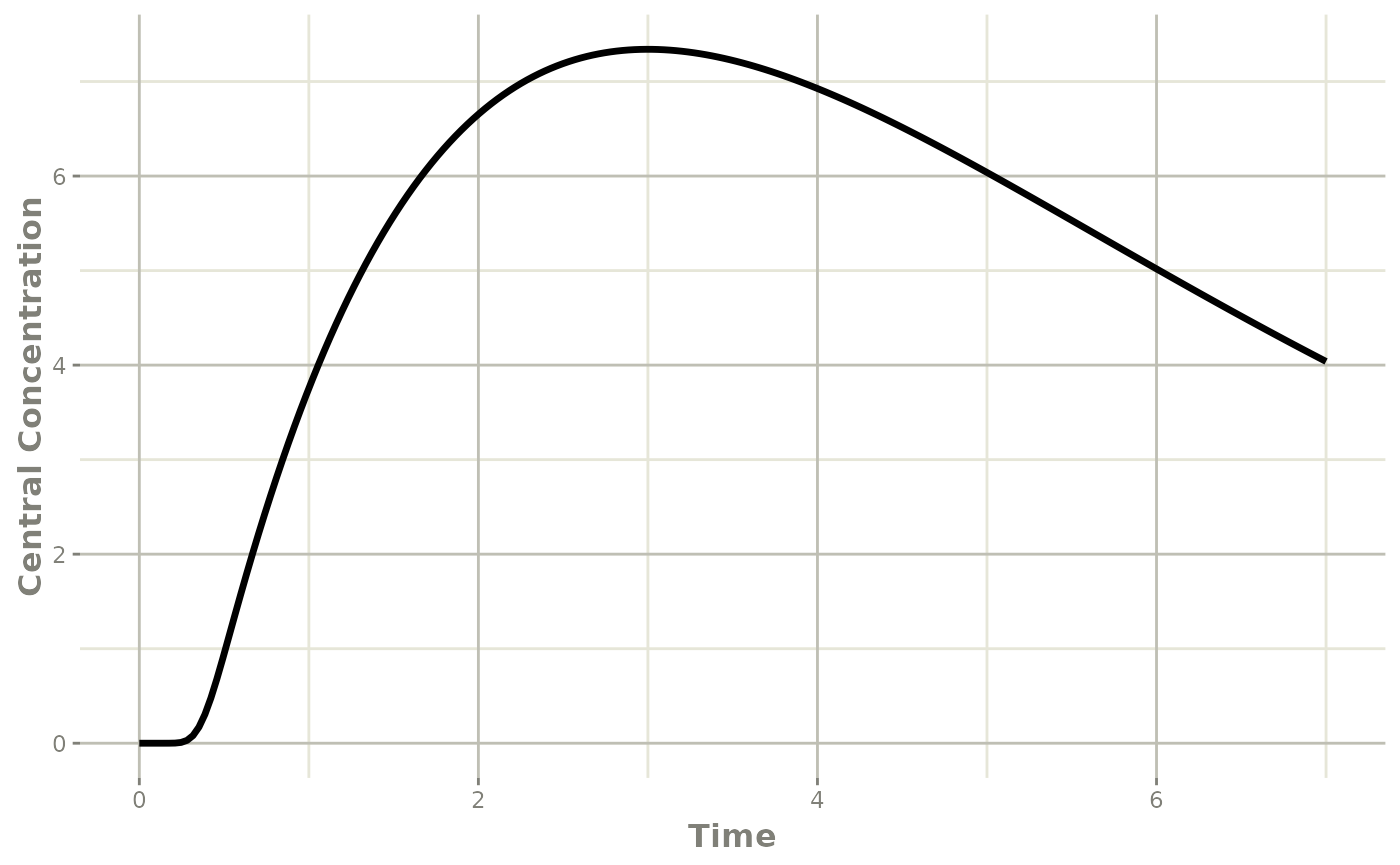Savic 2008 first introduced the idea of transit compartments being a mechanistic explanation of a a lag-time type phenomena. rxode2 has special handling of these models:

You can specify this in a similar manner as the original paper:

library(rxode2)
## rxode2 2.0.13.9000 using 1 threads (see ?getRxThreads)
##   no cache: create with rxCreateCache()
mod <- rxode2({
## Table 3 from Savic 2007
cl = 17.2 # (L/hr)
vc = 45.1 # L
ka = 0.38 # 1/hr
mtt = 0.37 # hr
bio=1
n = 20.1
k = cl/vc
ktr = (n+1)/mtt
## note that lgammafn is the same as lgamma in R.
d/dt(cen) = ka*depot-k*cen
})
## using C compiler: ‘gcc (Ubuntu 11.4.0-1ubuntu1~22.04) 11.4.0’
et <- eventTable()
et$add.sampling(seq(0, 7, length.out=200)) et$add.dosing(20, start.time=0, evid=7)

transit <- rxSolve(mod, et)

plot(transit, cen, ylab="Central Concentration")Another option is to specify the transit compartment function transit syntax. This specifies the parameters transit(number of transit compartments, mean transit time, bioavailability). The bioavailability term is optional.

The same model can be specified by:

mod <- rxode2({
## Table 3 from Savic 2007
cl = 17.2 # (L/hr)
vc = 45.1 # L
ka = 0.38 # 1/hr
mtt = 0.37 # hr
bio=1
n = 20.1
k = cl/vc
ktr = (n+1)/mtt
d/dt(depot) = transit(n,mtt,bio)-ka*depot
d/dt(cen) = ka*depot-k*cen
})
## using C compiler: ‘gcc (Ubuntu 11.4.0-1ubuntu1~22.04) 11.4.0’
et <- eventTable();
et$add.sampling(seq(0, 7, length.out=200)); et$add.dosing(20, start.time=0, evid=7);

transit <- rxSolve(mod, et)

plot(transit, cen, ylab="Central Concentration")A couple of things to keep in mind when using this approach:

• This approach implicitly assumes that the absorption through the transit compartment is completed before the next dose begins

• Different types of doses (ie bolus/infusion) to the compartment affect the time after dose calculation (tad) which is used in the transit compartment calculation. These (therefore) are not currently supported. The most stable way is to use tad(cmt) and podo(cmt), this way doses to other compartments do not affect the transit compartment calculation.

• Internally, the transit syntax uses either the currently defined cmt d/dt(cmt)=transit(...), or cmt. If the transit compartment is used outside of a d/dt() (not recommended), the cmt that is used is the last d/dt(cmt) defined it the model. This also means compartments do not affect one another (ie a oral, transit compartment drug dosed immediately with an IV infusion)